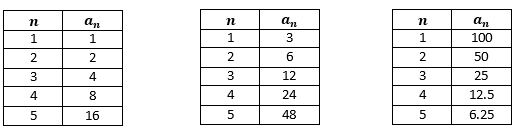# Exponents and Exponential Functions

## Objective

Write explicit rules for geometric sequences and translate between explicit and recursive formulas.

## Common Core Standards

### Core Standards

?

• F.BF.A.2 — Write arithmetic and geometric sequences both recursively and with an explicit formula, use them to model situations, and translate between the two forms. Modeling is best interpreted not as a collection of isolated topics but in relation to other standards. Making mathematical models is a Standard for Mathematical Practice, and specific modeling standards appear throughout the high school standards indicated by a star symbol (★). The star symbol sometimes appears on the heading for a group of standards; in that case, it should be understood to apply to all standards in that group.

• F.IF.A.3 — Recognize that sequences are functions, sometimes defined recursively, whose domain is a subset of the integers. For example, the Fibonacci sequence is defined recursively by f(0) = f(1) = 1, f(n+1) = f(n) + f(n-1) for n ≥ 1.

• F.LE.A.2 — Construct linear and exponential functions, including arithmetic and geometric sequences, given a graph, a description of a relationship, or two input-output pairs (include reading these from a table).

?

• 8.F.B.4

## Criteria for Success

?

1. Write explicit rules for geometric sequences and use them to find specific terms in the sequence.
2. Write recursive formulas for geometric sequences given an explicit formula, and vice versa.
3. Understand that the graph of a geometric sequence, written as a function, is an exponential function.

## Tips for Teachers

?

Use technology to show the graphs of geometric functions along with their explicit formulas. This is a preview of the work that students will do in upcoming lessons on exponential growth and decay.

## Anchor Problems

?

### Problem 1

The formula for an explicit rule for a geometric sequence is given by ${a_n=a_1(r)^{n-1}}$, where $r$ is the common ratio and $n$ is the term number.

Write an explicit formula for the sequence shown in each table.#### Guiding Questions

Create a free account or sign in to access the Guiding Questions for this Anchor Problem.

### Problem 2

Act 1: Watch the Act 1 video of the “Incredible Shrinking Dollar.” If Dan shrinks the dollar nine times like this, how big will it be? Will you still be able to see it?

a. Give a guess you know is too big.

b. Give a guess you know is too small.

c. What information would help solve this problem?

Act 2: Share the dimensions of the dollar bill provided on the website

d. Do the changing lengths of the dollar bill follow a geometric sequence pattern or an arithmetic sequence pattern?

e. Write an explicit formula to represent the length of the dollar bill as it shrinks.

Act 3: Watch Act 3 video.

f. Calculate the actual length of the dollar bill after Dan shrank it nine times.

#### Guiding Questions

Create a free account or sign in to access the Guiding Questions for this Anchor Problem.

#### References

Dan Meyer's Three-Act Math Incredible Shrinking Dollar

Incredible Shrinking Dollar by Dan Meyer is licensed under the CC BY 3.0 license. Accessed Feb. 27, 2018, 8:56 a.m..

Modified by Fishtank Learning, Inc.

## Problem Set

?

The following resources include problems and activities aligned to the objective of the lesson that can be used to create your own problem set.

• Mathematics Vision Project: Secondary Mathematics One Module 1: Sequences1.7 "Set", 1.8 "What Comes Next? What Comes Later?", "Ready", "Set", 1.9 "Go"
• MathTalks Math Talks(Switch to Pattern Talks to find arithmetic sequences shown as visual patterns)
• Kuta Software Free Algebra 2 Worksheets Geometric Sequences

## Target Task

?

Given the following information, determine the explicit equation for each geometric sequence.

a.   ${f(1)=8}$, common ratio ${r=2}$

b.   ${f(1)=4,f(n)=3f(n-1)}$

c.   ${f(n)=4f(n-1);f(1)={5\over3}}$

Which geometric sequence above has the greatest value at ${f(100)}$?